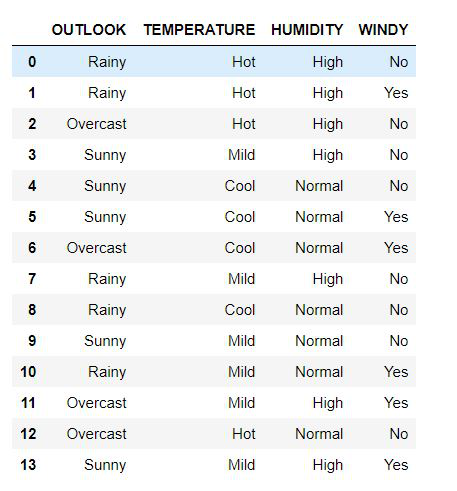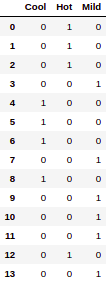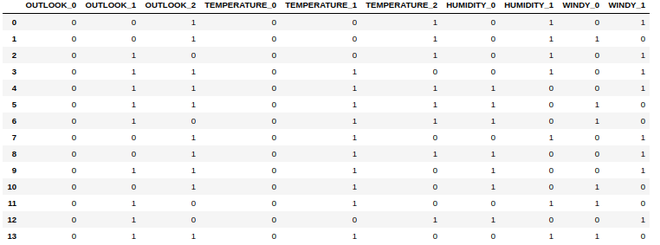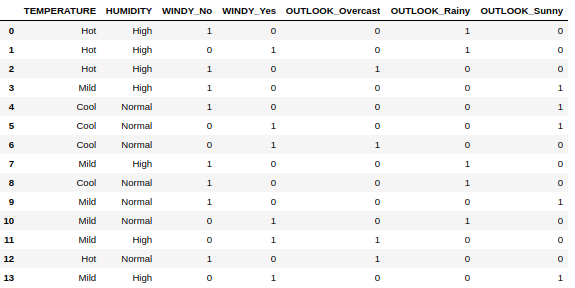Related Articles
Convert A Categorical Variable Into Dummy Variables
• Last Updated : 11 Dec, 2020

All the statistical and machine learning models are built on the foundation of data. A grouped or composite entity holding the relevant to a particular problem together is called a data set. These data sets are composed of Independent Variables or the features and the Dependent Variables or the Labels. All of these variables can be classified into two types of data: Quantitative and Categorical.

In this article, we are going to deal with the various methods to convert Categorical Variables into Dummy Variables which is an essential part of data pre-processing, which in itself is an integral part of the Machine Learning or Statistical Model. The categorical variables can be further subdivided into the following categories :

• Binary or Dichotomous is essentially the variables that can have only two outcomes such as Win/Lose, On/Off, and so on.
• Nominal Variables are used to represent groups with no particular ranking such as colors, brands, and so on.
• Ordinal Variables represent groups with a specified ranking order such as Winners of a race, App Ratings to name a few.

Dummy Variables act as indicators of the presence or absence of a category in a Categorical Variable. The usual convention dictates that 0 represents absence while 1 represents presence. The conversion of Categorical Variables into Dummy Variables leads to the formation of the two-dimensional binary matrix where each column represents a particular category. The following example will further clarify the process of conversion.

Data set containing categorical variable:

Data set containing a dummy variable :

Explanation:

The above data set comprises four categorical columns: OUTLOOK, TEMPERATURE, HUMIDITY, WINDY.

Let’s consider the column WINDY which is composed of two categories: YES and NO. So, in the data set that contains the Dummy Variables, the column WINDY is replaced by two columns which each represent the categories: YES and NO. Now comparing the rows of the columns YES and NO with WINDY, we mark 0 for YES where it is absent and 1 where it is present. The same is done for column NO. This methodology is adopted for all the categorical columns. The important thing to notice is that each categorical column is replaced by the number of unique categories it has in the data set containing dummy variables.

We are going to be exploring three approaches to convert Categorical Variables into Dummy Variables in this article.

These approaches are as follows:

1. Using the LabelBinarizer from sklearn
2. Using BinaryEncoder from category_encoders
3. Using the get_dummies() function of the pandas library

### Creating the data set:

The first step is creating the data set. This data set comprises 4 categorical columns which go by the name of OUTLOOK, TEMPERATURE, HUMIDITY, WINDY. The following is the code for the creation of the data set. We make this data set using the pandas.DataFrame() and dictionary.

## Python3

 `# code to create the dataset`` ` `# importing the libraries``import` `pandas as pd`` ` `# creating the dictionary``dictionary ``=` `{``'OUTLOOK'``: [``'Rainy'``, ``'Rainy'``,``                          ``'Overcast'``, ``'Sunny'``,``                          ``'Sunny'``, ``'Sunny'``,``                          ``'Overcast'``, ``'Rainy'``,``                          ``'Rainy'``, ``'Sunny'``,``                          ``'Rainy'``, ``'Overcast'``,``                          ``'Overcast'``, ``'Sunny'``],``              ``'TEMPERATURE'``: [``'Hot'``, ``'Hot'``, ``'Hot'``,``                              ``'Mild'``, ``'Cool'``,``                              ``'Cool'``, ``'Cool'``,``                              ``'Mild'``, ``'Cool'``,``                              ``'Mild'``, ``'Mild'``,``                              ``'Mild'``, ``'Hot'``, ``'Mild'``],``              ``'HUMIDITY'``: [``'High'``, ``'High'``, ``'High'``,``                           ``'High'``, ``'Normal'``, ``'Normal'``,``                           ``'Normal'``, ``'High'``, ``'Normal'``,``                           ``'Normal'``, ``'Normal'``, ``'High'``,``                           ``'Normal'``, ``'High'``],``              ``'WINDY'``: [``'No'``, ``'Yes'``, ``'No'``, ``'No'``, ``'No'``,``                        ``'Yes'``, ``'Yes'``, ``'No'``, ``'No'``,``                        ``'No'``, ``'Yes'``, ``'Yes'``, ``'No'``,``                        ``'Yes'``]}`` ` `# converting the dictionary to DataFrame``df ``=` `pd.DataFrame(dictionary)`` ` `display(df)`

Output:The above is the data set that we will be using for the approaches ahead.

### Approach 1:

Using this approach, we use LabelBinarizer from sklearn which converts one categorical column to a data frame with dummy variables at a time. This data frame can then be appended to the main data frame in the case of there being more than one Categorical column.

## Python3

 `# importing the libraries``from` `sklearn.preprocessing ``import` `LabelBinarizer`` ` `# creating a copy of the``# original data frame``df1 ``=` `df.copy()`` ` `# creating an object ``# of the LabelBinarizer``label_binarizer ``=` `LabelBinarizer()`` ` `# fitting the column ``# TEMPERATURE to LabelBinarizer``label_binarizer_output ``=` `label_binarizer.fit_transform( df1[``'TEMPERATURE'``])`` ` `# creating a data frame from the object``result_df ``=` `pd.DataFrame(label_binarizer_output,``                         ``columns ``=` `label_binarizer.classes_)`` ` `display(result_df)`

Output:Conversion of TEMPERATURE column

Similarly, we can transform other categorical columns as well.

### Approach 2:

Using the BinaryEncoder from the category_encoders library. Using this approach we can convert multiple categorical columns into dummy variables in a single go.

category_encoders: The category_encoders is a Python library developed under the scikit-learn-transformers library. The primary objective of this library is to convert categorical variables into quantifiable numeric variables. There are various advantages of this library such as being readily compatible with the sklearn transformers which allow them to be readily trained and stored in serializable files such as pickle for later use. This library works great in working with data frames as well which is of great use while dealing with machine learning and statistical models. It provides a great range of methods for the conversion from categorical to numeric variables as well which can be categorized into Supervised and Unsupervised.

For installation run this command into the terminal:

`pip install category_encoders`

For conda:

`conda install -c conda-forge category_encoders`

Code:

## Python3

 `# importing the libraries``import` `category_encoders as cat_encoder`` ` `# creating a copy of the original data frame``df2 ``=` `df.copy()`` ` `# creating an object BinaryEncoder``# this code calls all columns``# we can specify specific columns as well``encoder ``=` `cat_encoder.BinaryEncoder(cols ``=` `df2.columns)`` ` `# fitting the columns to a data frame``df_category_encoder ``=` `encoder.fit_transform( df2 )`` ` `display(df_category_encoder)`

Output:Data Frame created from all the Categorical Columns

### Approach 3:

Under this approach, we deploy the simplest way to perform the conversion of all possible Categorical Columns in a data frame to Dummy Columns by using the get_dummies() method of the pandas library.

We can either specify the columns to get the dummies by default it will convert all the possible categorical columns to their dummy columns.

## Python3

 `# importing the libraries``import` `pandas as pd`` ` `# creating a copy of the original data frame``df3 ``=` `df.copy()`` ` `# calling the get_dummies method``# the first parameter mentions the``# the name of the data frame to store the``# new data frame in``# the second parameter is the list of``# columns which if not mentioned``# returns the dummies for all``# categorical columns``df3 ``=` `pd.get_dummies(df3,``                     ``columns ``=` `[``'WINDY'``, ``'OUTLOOK'``])`` ` `display(df3)`

Output:Using the get_dummies() for the columns WINDY and OUTLOOKMy Personal Notes arrow_drop_up